JEE  >  Test: MCQs (One or More Correct Option): Limits, Continuity and Differentiability | JEE Advanced

# Test: MCQs (One or More Correct Option): Limits, Continuity and Differentiability | JEE Advanced

Test Description

## 25 Questions MCQ Test Maths 35 Years JEE Main & Advanced Past year Papers | Test: MCQs (One or More Correct Option): Limits, Continuity and Differentiability | JEE Advanced

Test: MCQs (One or More Correct Option): Limits, Continuity and Differentiability | JEE Advanced for JEE 2022 is part of Maths 35 Years JEE Main & Advanced Past year Papers preparation. The Test: MCQs (One or More Correct Option): Limits, Continuity and Differentiability | JEE Advanced questions and answers have been prepared according to the JEE exam syllabus.The Test: MCQs (One or More Correct Option): Limits, Continuity and Differentiability | JEE Advanced MCQs are made for JEE 2022 Exam. Find important definitions, questions, notes, meanings, examples, exercises, MCQs and online tests for Test: MCQs (One or More Correct Option): Limits, Continuity and Differentiability | JEE Advanced below.
Solutions of Test: MCQs (One or More Correct Option): Limits, Continuity and Differentiability | JEE Advanced questions in English are available as part of our Maths 35 Years JEE Main & Advanced Past year Papers for JEE & Test: MCQs (One or More Correct Option): Limits, Continuity and Differentiability | JEE Advanced solutions in Hindi for Maths 35 Years JEE Main & Advanced Past year Papers course. Download more important topics, notes, lectures and mock test series for JEE Exam by signing up for free. Attempt Test: MCQs (One or More Correct Option): Limits, Continuity and Differentiability | JEE Advanced | 25 questions in 50 minutes | Mock test for JEE preparation | Free important questions MCQ to study Maths 35 Years JEE Main & Advanced Past year Papers for JEE Exam | Download free PDF with solutions
 1 Crore+ students have signed up on EduRev. Have you?
*Multiple options can be correct
Test: MCQs (One or More Correct Option): Limits, Continuity and Differentiability | JEE Advanced - Question 1

### If x+ | y | = 2y , then y as a function of x is

Detailed Solution for Test: MCQs (One or More Correct Option): Limits, Continuity and Differentiability | JEE Advanced - Question 1

Given that x + | y | = 2y
If y < 0 then x – y = 2y
⇒ y = x/3 ⇒ x < 0
If y = 0 then x = 0. If y > 0 then x + y = 2y
⇒ y = x ⇒ x > 0

Thus we can define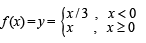Continuity at x = 0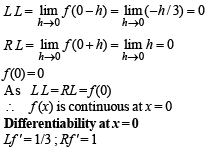As Lf ' ≠ Rf ' ⇒ f (x) is not differentiable at x = 0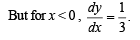*Multiple options can be correct
Test: MCQs (One or More Correct Option): Limits, Continuity and Differentiability | JEE Advanced - Question 2

###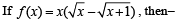Detailed Solution for Test: MCQs (One or More Correct Option): Limits, Continuity and Differentiability | JEE Advanced - Question 2

We have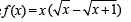Let us check differentiability of f (x) at x = 0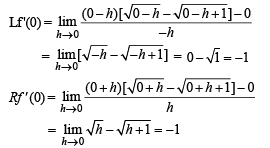Since Lf ' (0) = Rf ' (0)
∴ f is differentiable at x = 0.

*Multiple options can be correct
Test: MCQs (One or More Correct Option): Limits, Continuity and Differentiability | JEE Advanced - Question 3

### The function f (x) = 1 + | sin x | is

Detailed Solution for Test: MCQs (One or More Correct Option): Limits, Continuity and Differentiability | JEE Advanced - Question 3

The graph of f (x) = 1 + | sin x | is as shown in the fig.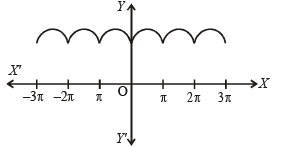From graph it is clear that function is continuous every where but not differentiable at integral multiples of π (∴ at these points curve has sharp turnings)

*Multiple options can be correct
Test: MCQs (One or More Correct Option): Limits, Continuity and Differentiability | JEE Advanced - Question 4

Let [x] denote the greatest integer less than or equal to x. If f(x) = [x sin π x], then f(x) is

Detailed Solution for Test: MCQs (One or More Correct Option): Limits, Continuity and Differentiability | JEE Advanced - Question 4

We have, for  – 1 < x < 1 ⇒  0 < x sin π x < 1/2
∴ f (x) = [x sin π x] = 0
Also x sin p x becomes negative and numerically less than 1 when x is slightly greater than 1 and so by definition of [x],
f (x) = [x sin π x] = – 1 when 1 < x < 1 + h
Thus f (x) is constant and equal to 0 in the closed interval [– 1, 1] and so f (x) is continuous and differentiable in the open interval (– 1, 1).
At x = 1, f (x) is clearly discontinuous, since f (1– 0) = 0 and f (1 + 0) = – 1 and f (x) is non-differentiable at x = 1

*Multiple options can be correct
Test: MCQs (One or More Correct Option): Limits, Continuity and Differentiability | JEE Advanced - Question 5

The set of all points where the function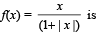differentiable, is

Detailed Solution for Test: MCQs (One or More Correct Option): Limits, Continuity and Differentiability | JEE Advanced - Question 5

The given function is,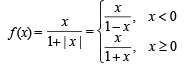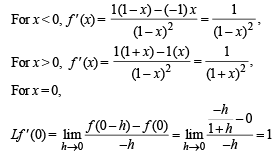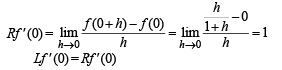⇒ f is differentiable at x = 0
Hence f is differentiable in (– ∞, ∞).

*Multiple options can be correct
Test: MCQs (One or More Correct Option): Limits, Continuity and Differentiability | JEE Advanced - Question 6

The function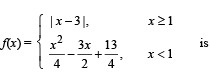Detailed Solution for Test: MCQs (One or More Correct Option): Limits, Continuity and Differentiability | JEE Advanced - Question 6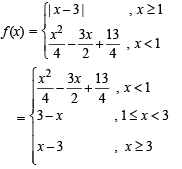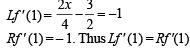∴ f is differentiable at x = 1 and hence continuous at x = 1.
Again,  Lf ' (3) = – 1 and Rf ' (3) = 1
⇒ Lf ' (3) ≠ Rf ' (3)
⇒ f is not differentiable at x = 3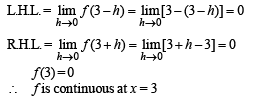*Multiple options can be correct
Test: MCQs (One or More Correct Option): Limits, Continuity and Differentiability | JEE Advanced - Question 7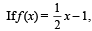then on the interval [0, π]

Detailed Solution for Test: MCQs (One or More Correct Option): Limits, Continuity and Differentiability | JEE Advanced - Question 7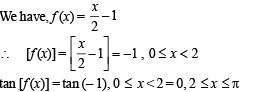∴ The function tan [f (x)] is discontinuous at x = 2.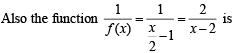discontinuous at x = 2.
Thus both the given functions tan [f (x)] as well as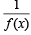are discontinuous on the interval [0, π].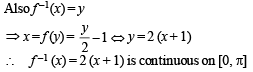*Multiple options can be correct
Test: MCQs (One or More Correct Option): Limits, Continuity and Differentiability | JEE Advanced - Question 8

The value of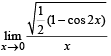Detailed Solution for Test: MCQs (One or More Correct Option): Limits, Continuity and Differentiability | JEE Advanced - Question 8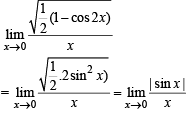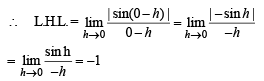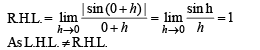∴ The given limit does not exist.

*Multiple options can be correct
Test: MCQs (One or More Correct Option): Limits, Continuity and Differentiability | JEE Advanced - Question 9

The following functions are continuous on (0, π).

Detailed Solution for Test: MCQs (One or More Correct Option): Limits, Continuity and Differentiability | JEE Advanced - Question 9

On (0, π)
(a) tan x = f (x)
We know that tan x is discontinuous at x = π/2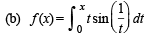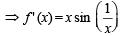which exists on (0, π)
∴ f (x), being differentiable, is continuous on (0, π).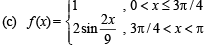Clearly f (x) is continuous on (0, π) except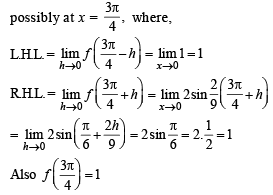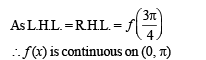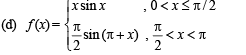Here f (x) will be continuous on (0, π) if it is continuous at x = π/2.
At x =π/2,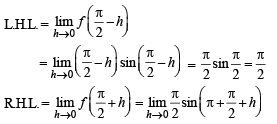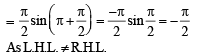∴ f (x) is not continuous on (0, π).

*Multiple options can be correct
Test: MCQs (One or More Correct Option): Limits, Continuity and Differentiability | JEE Advanced - Question 10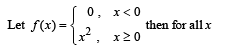Detailed Solution for Test: MCQs (One or More Correct Option): Limits, Continuity and Differentiability | JEE Advanced - Question 10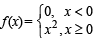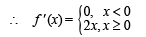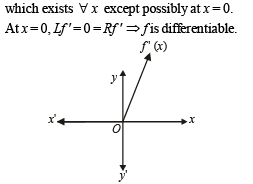From graph of f ' (x), it is clear that f ' (x) is continuous but not differentiable at x = 0.

*Multiple options can be correct
Test: MCQs (One or More Correct Option): Limits, Continuity and Differentiability | JEE Advanced - Question 11

Let g(x) = x f(x), where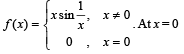Detailed Solution for Test: MCQs (One or More Correct Option): Limits, Continuity and Differentiability | JEE Advanced - Question 11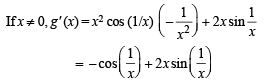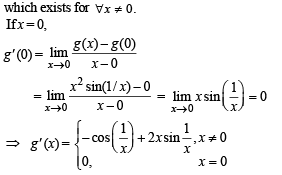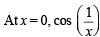is not continuous, therefore g' (x) is not continuous at x = 0.
At x = 0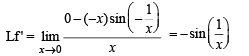which does not exist.
∴ f is not differentiable at x = 0.

*Multiple options can be correct
Test: MCQs (One or More Correct Option): Limits, Continuity and Differentiability | JEE Advanced - Question 12

The function f(x) = max {(1 – x), (1 + x), 2}, x ∈ ( -∞,∞) is

Detailed Solution for Test: MCQs (One or More Correct Option): Limits, Continuity and Differentiability | JEE Advanced - Question 12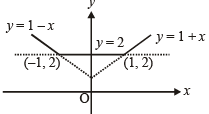From graph it is clear that f (x) is continuous every where and also differentiable everywhere except at  x = 1 and – 1.

*Multiple options can be correct
Test: MCQs (One or More Correct Option): Limits, Continuity and Differentiability | JEE Advanced - Question 13

Let h(x) = min {x, x2}, for every real number of x, Then

Detailed Solution for Test: MCQs (One or More Correct Option): Limits, Continuity and Differentiability | JEE Advanced - Question 13

From the figure it is clear that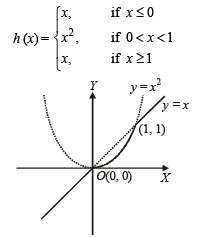From the graph it is clear that h is continuous for all x ∈ R , h' (x) = 1 for all x > 1 and h is not differentiable at x = 0 and 1.

*Multiple options can be correct
Test: MCQs (One or More Correct Option): Limits, Continuity and Differentiability | JEE Advanced - Question 14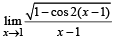Detailed Solution for Test: MCQs (One or More Correct Option): Limits, Continuity and Differentiability | JEE Advanced - Question 14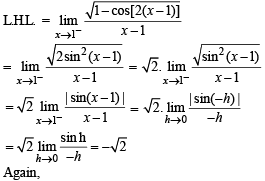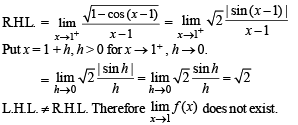*Multiple options can be correct
Test: MCQs (One or More Correct Option): Limits, Continuity and Differentiability | JEE Advanced - Question 15

If f(x) = min {1, x2, x3}, then

Detailed Solution for Test: MCQs (One or More Correct Option): Limits, Continuity and Differentiability | JEE Advanced - Question 15

From graph, f (x) is continuous everywhere but not differentiable at x = 1.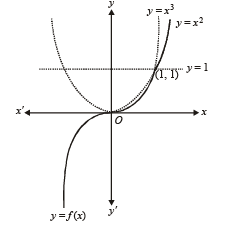*Multiple options can be correct
Test: MCQs (One or More Correct Option): Limits, Continuity and Differentiability | JEE Advanced - Question 16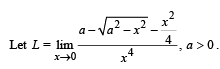If L is finite, then

Detailed Solution for Test: MCQs (One or More Correct Option): Limits, Continuity and Differentiability | JEE Advanced - Question 16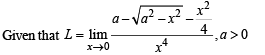and L is finite.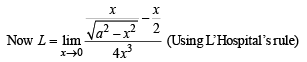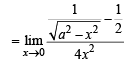∴ L is finite, limiting value of numerator zero which is so when should be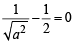i.e. a = 2 (∴ a > 0)

Applying L ‘Hospital’s rule again, we ge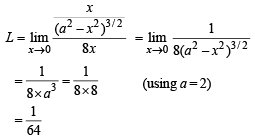*Multiple options can be correct
Test: MCQs (One or More Correct Option): Limits, Continuity and Differentiability | JEE Advanced - Question 17

Let f : R → R be a function such that  f (x + y) =  f (x) +  f (y),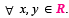If  f (x) is differentiable at x = 0, then

Detailed Solution for Test: MCQs (One or More Correct Option): Limits, Continuity and Differentiability | JEE Advanced - Question 17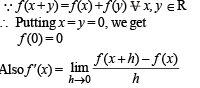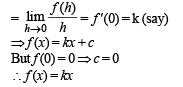Which is continuous and differentiable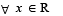∴ b and c are the correct options.

*Multiple options can be correct
Test: MCQs (One or More Correct Option): Limits, Continuity and Differentiability | JEE Advanced - Question 18Detailed Solution for Test: MCQs (One or More Correct Option): Limits, Continuity and Differentiability | JEE Advanced - Question 18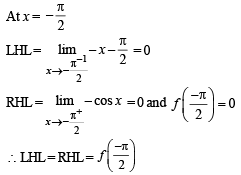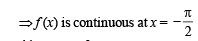Also at x  = 0
Lf '(0) = sin 0 = 0; Rf '(0) = 1 – 0 = 1
∴ Lf '(0) ≠ Rf '(0)
⇒ f is not differentiable at x = 0
At x = 1
Lf '(1) = R'f (1) ⇒ f is differentiable at x = 1.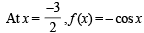which is differentiable.
∴ All four options are correct.

*Multiple options can be correct
Test: MCQs (One or More Correct Option): Limits, Continuity and Differentiability | JEE Advanced - Question 19

For every integer n, let an and bn be real numbers. Let function f : IR → IR be given by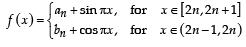for all integers n. If f is continuous, then which of the following hold(s) for all n ?

Detailed Solution for Test: MCQs (One or More Correct Option): Limits, Continuity and Differentiability | JEE Advanced - Question 19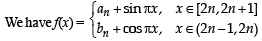As f is continuous for all n
∴At x = 2n, LHL = RHL = f (2n)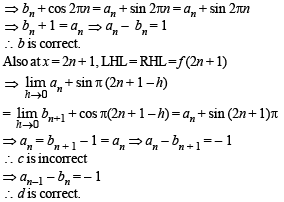*Multiple options can be correct
Test: MCQs (One or More Correct Option): Limits, Continuity and Differentiability | JEE Advanced - Question 20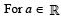(the set of all real numbers), a ≠ –1,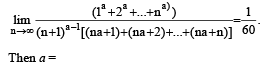Detailed Solution for Test: MCQs (One or More Correct Option): Limits, Continuity and Differentiability | JEE Advanced - Question 20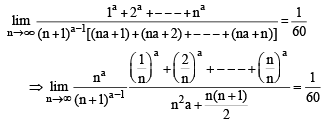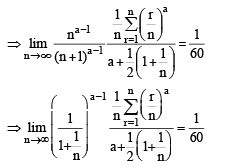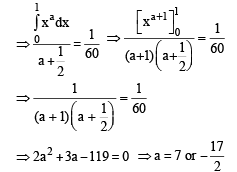*Multiple options can be correct
Test: MCQs (One or More Correct Option): Limits, Continuity and Differentiability | JEE Advanced - Question 21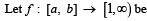a continuous function and let g :  R → R be defined as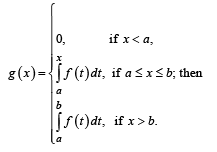Detailed Solution for Test: MCQs (One or More Correct Option): Limits, Continuity and Differentiability | JEE Advanced - Question 21

g(x) may be discontinuous at x = a or x = b.
Let us check the continuity of g(x) at x = a and x = b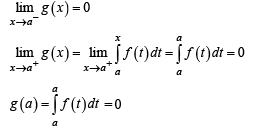∴ g (x) is continuous at x = a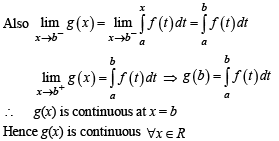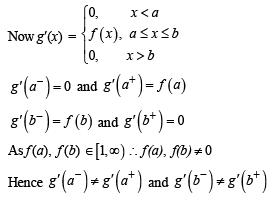∴ g is not differentiable at a and b

*Multiple options can be correct
Test: MCQs (One or More Correct Option): Limits, Continuity and Differentiability | JEE Advanced - Question 22

For every pair of continuous functions f, g : [0, 1] → R such that max {f ( x) :x ∈[ 0,1]} = max {g (x) :x ∈[ 0,1]} , the correct statement(s) is (are):

Detailed Solution for Test: MCQs (One or More Correct Option): Limits, Continuity and Differentiability | JEE Advanced - Question 22

Let f and g be maximum at c1 and c2 respectively,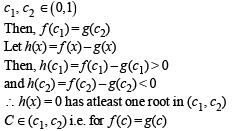which shows that (a) and (d) are correct.

*Multiple options can be correct
Test: MCQs (One or More Correct Option): Limits, Continuity and Differentiability | JEE Advanced - Question 23

Let g : R → R be a differentiable function with g(0) = 0, g'(0) = 0 and g'(1) ≠ 0. Let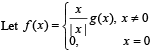and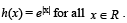Let (foh)(x) denote f(h(x)) and (hof)(x) denote h(f(x)). Then which of the following is (are) true?

Detailed Solution for Test: MCQs (One or More Correct Option): Limits, Continuity and Differentiability | JEE Advanced - Question 23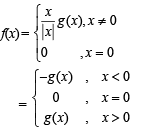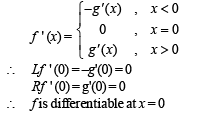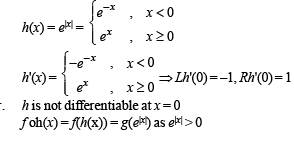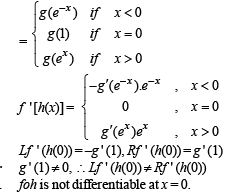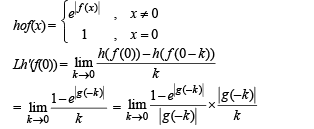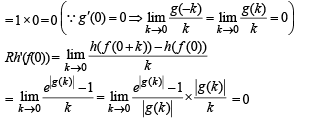∴ hof is differentiable at x = 0.

*Multiple options can be correct
Test: MCQs (One or More Correct Option): Limits, Continuity and Differentiability | JEE Advanced - Question 24

Let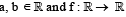be defined by f (x) = a cos (|x3 –x|) + b |x| sin (|x3 +x|).

Then f is

Detailed Solution for Test: MCQs (One or More Correct Option): Limits, Continuity and Differentiability | JEE Advanced - Question 24

f(x) = a cos3(|x3 –x|) + b |x| sin (|x3 + x|)
(a) If a = 0, b = 1
⇒ f(x) = |x| sin |x3 + x|
= x sin (x3 + x), x ∈ R
∴ f is differentiable every where.
(b), (c) If a = 1, b = 0 ⇒ f(x) = cos3 (|x3 – x|)
= cos3(x3 – x)
which is differentiable every where.
(d) when a = 1, b = 1, f(x) = cos(x3 – x) + x sin (x3 + x) which is differentiable at x = 1
∴ Only a and b are the correct options.

*Multiple options can be correct
Test: MCQs (One or More Correct Option): Limits, Continuity and Differentiability | JEE Advanced - Question 25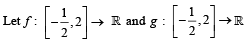be fun ctions defined by f (x) = [x2–3] and g(x) = |x| f (x) + |4x–7 | f (x), where [y] denotes the greatest integer less than or equal to y for y ∈ R. Then

Detailed Solution for Test: MCQs (One or More Correct Option): Limits, Continuity and Differentiability | JEE Advanced - Question 25

f(x) = [x2 – 3] is discontinuous at all integral points in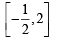∴ f is discontinuous exactly at four points inAlso g (x) = (|x| + |4x- 7|)f (x)

Here f is not differentiable at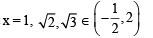and |x| + |4x – 7| is not differentiable at 0 and 7/4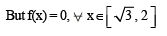∴ g(x) becomes differentiable at x = 7/4

Hence g(x) is non-differentiable at four points i.e.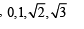## Maths 35 Years JEE Main & Advanced Past year Papers

111 docs|70 tests
 Use Code STAYHOME200 and get INR 200 additional OFF Use Coupon Code
Information about Test: MCQs (One or More Correct Option): Limits, Continuity and Differentiability | JEE Advanced Page
In this test you can find the Exam questions for Test: MCQs (One or More Correct Option): Limits, Continuity and Differentiability | JEE Advanced solved & explained in the simplest way possible. Besides giving Questions and answers for Test: MCQs (One or More Correct Option): Limits, Continuity and Differentiability | JEE Advanced, EduRev gives you an ample number of Online tests for practice

## Maths 35 Years JEE Main & Advanced Past year Papers

111 docs|70 tests